# Diagram(2)

(diff) ← Older revision | Latest revision (diff) | Newer revision → (diff)

Letand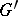be directed graphs (also called oriented graphs, diagram schemes or pre-categories; cf. also Graph, oriented). A diagram of shape (also called a diagram of type)inis a morphism of graphs; i.e. ifandare given by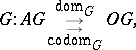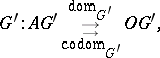(here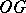and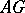denote, respectively, a set of objects and a set of arrows of), then a morphismis a pair of mappings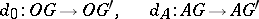with,.

A diagram is called finite if its shape is a finite graph, i.e.andare finite sets. A diagram in a category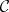is defined as a diagram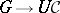, where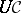denotes the underlying graph of(with the same objects and arrows, forgetting which arrows are composites and which are identities).

Every functor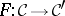is also a diagram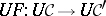between the corresponding graphs. This observation defines the forgetful functor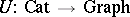from small categories to small graphs (cf. also Functor).

Let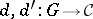be two diagrams of the same shapein the same category. A morphism betweenandis a mapping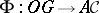that carries each objectof the graphto an arrow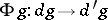, such that for any arrow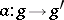ofthe diagram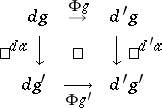commutes.

All diagrams of the shapeinand all morphisms between them form a category.

Let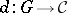be a diagram in the categoryand let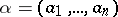be a finite sequence of arrows of the graphwith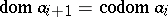,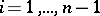. Put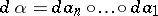. A diagramis called commutative iffor any finite sequence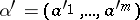inwith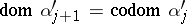,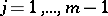,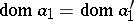,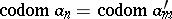.

A sequence is a diagram, whereis of the form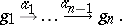The corresponding diagram is represented by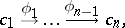whereare objects andare arrows of.

A triangle diagram in the categoryis a diagram with shape graph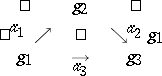and is represented asCommutativity means that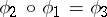.

A quadratic diagram (also called a square diagram) incorresponds to the graphand is represented asCommutativity means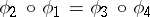.

How to Cite This Entry:
Diagram(2). Encyclopedia of Mathematics. URL: http://encyclopediaofmath.org/index.php?title=Diagram(2)&oldid=12484
This article was adapted from an original article by T. Datuashvili (originator), which appeared in Encyclopedia of Mathematics - ISBN 1402006098. See original article